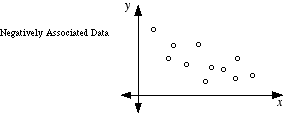index: click on a letter A B C D E F G H I J K L M N O P Q R S T U V W X Y Z A to Z index index: subject areas numbers & symbols sets, logic, proofs geometry algebra trigonometry advanced algebra & pre-calculus calculus advanced topics probability & statistics real world applications multimedia entrieswww.mathwords.com about mathwords website feedback

 Negatively Associated Data A relationship in paired data in which one variable's values tend to increase when the other decreases, and vice-versa. In a scatterplot, negatively associated data tend to follow a pattern from the upper left to the lower right. Negatively associated data have a negative correlation coefficient.See also